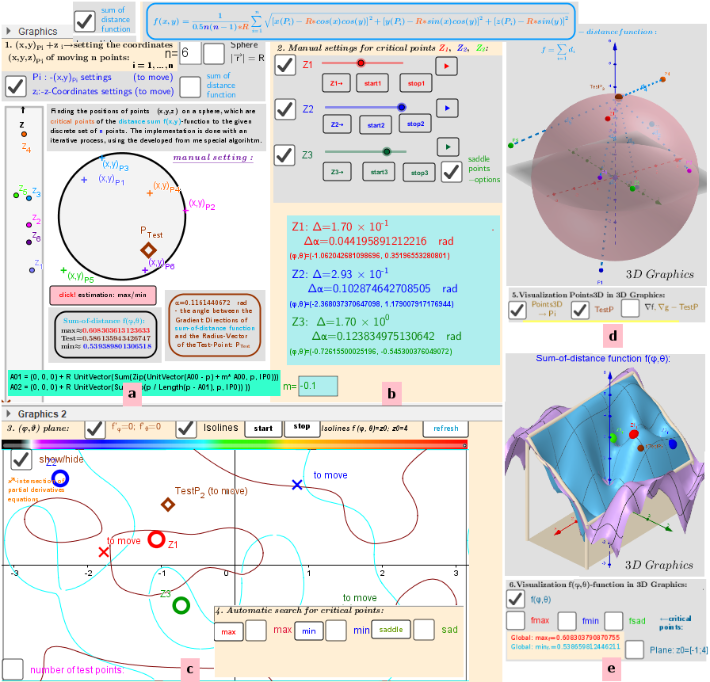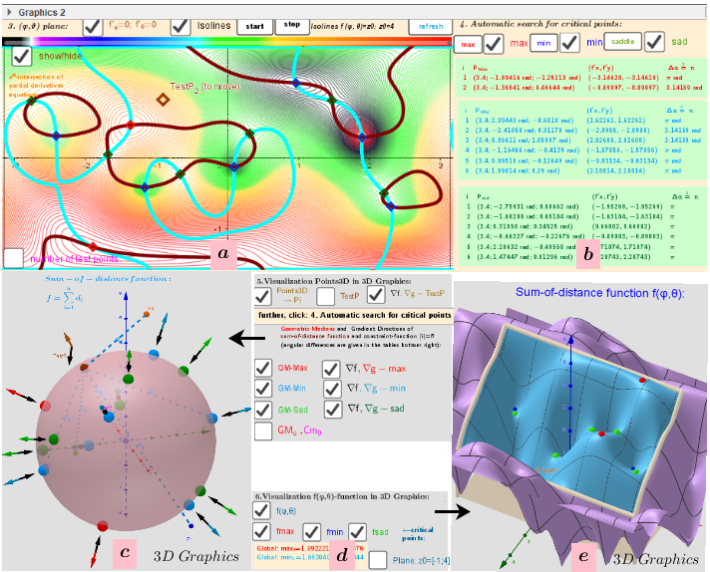# Example 2 of Applet in which 6 moving points in three-dimensional space "induce" 14 geometric medians on a sphere.

Let Pi=(xi,yi,zi) n moving points in ℝ³ (lP:={P1,P2,...,Pn}). I want to find  the points P=(x,y,z) on the surface of the sphere -S (radius R) that are critical (relative min/max or saddle points at (x,y,z)) of a function f(x,y,z) is the sum of the distances from P to the all points from lP. Critical points can be found using Lagrange multipliersas finding the Extreme values of the function f(x,y,z) subject to a g(x,y,z)=0 (constraining equation: g(x,y,z)=x2+y2+z2-R2). There is a system of equations: ∇f(x,y,z)= λ∇g(x,y,z). A local optimum occurs when ∇f(x,y,z) and ∇g(x,y,z) are parallel, and so ∇f is some multiple of ∇g. This applet is used to study the distribution of geometric medians on a sphere of radius R, „induces“ by the discrete sample of 6 movable points in the 3-D space. Description is in https://www.geogebra.org/m/y8dnkeuu.Fig.1 Settings and control panels, observation windows. a) -Settings and d) -relative position of the the "influence" points Pi around the sphere. b) -Manual settings for finding critical points f (φ, θ) and determining the coefficient m for the saddle point search algorithm. c) -(φ;θ) -plane of the angular coordinates of points on the sphere: Z1, Z2, Z3 -moving points (from b) of iterative solution search; f'_φ=0; f'_θ=0 -implicit functions of equations , their intersection are solutions of the Lagrange equations. e) -graph of the distance sum function f (φ, θ).Fig.2 Results of explorations. a) -Isolines and intersecting the implicit function equations of zeroing partial derivatives. b) - Automatic search for critical points; their values. c) -Distribution of points Pi, test Point, Max/min/saddle -Critical points on a sphere. Vectors ∇f and ∇g at these points. d) -settings and e) -graph of the Two-variable function f(φ,θ) over a rectangular region: - π ≤φ ≤ π; -π/2≤θ≤π/2.